IMO Shortlist 2009 problem C8

Kvaliteta:
Avg: 0,0
Težina:
Avg: 9,0
For any integer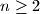$n\geq 2$, we compute the integer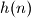$h(n)$ by applying the following procedure to its decimal representation. Let$r$ be the rightmost digit of$n$.
If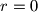$r=0$, then the decimal representation of$h(n)$ results from the decimal representation of$n$ by removing this rightmost digit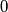$0$.If$1\leq r \leq 9$ we split the decimal representation of$n$ into a maximal right part$R$ that solely consists of digits not less than$r$ and into a left part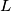$L$ that either is empty or ends with a digit strictly smaller than$r$. Then the decimal representation of$h(n)$ consists of the decimal representation of$L$, followed by two copies of the decimal representation of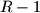$R-1$. For instance, for the number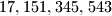$17,151,345,543$, we will have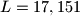$L=17,151$,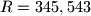$R=345,543$ and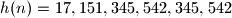$h(n)=17,151,345,542,345,542$.Prove that, starting with an arbitrary integer$n\geq 2$, iterated application of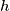$h$ produces the integer$1$ after finitely many steps.

Proposed by Gerhard Woeginger, Austria
Izvor: Međunarodna matematička olimpijada, shortlist 2009